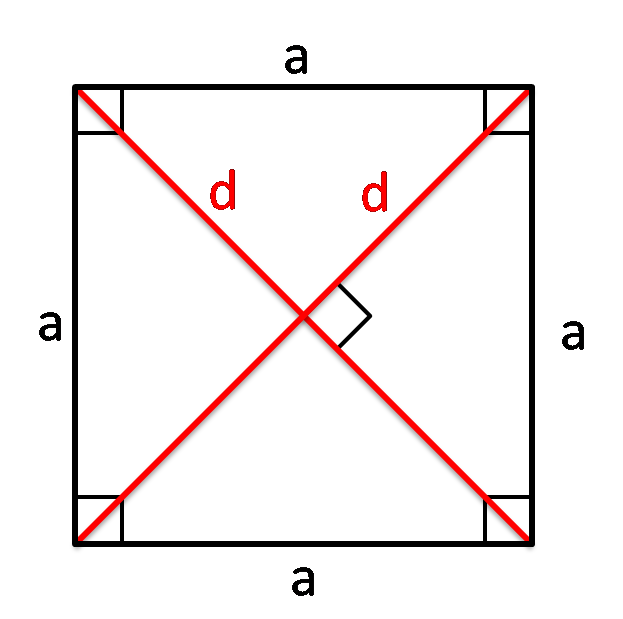# Diagonal of a Square Calculator

Created by Hanna Pamuła, PhD candidate
Reviewed by Bogna Szyk
Last updated: Feb 15, 2022

Find out the length of square diagonal with our diagonal of a square calculator. Check out below how to find the diagonal of a square formula or simply give our tool a try - you won't be disappointed.

## Diagonal of squareDiagonal is a line segment that joins two non-neighboring vertices. Each quadrilateral has two diagonals, a square too. Its diagonals are:

• equal in length
• perpendicular bisectors of each other
• bisectors of square angles

Each diagonal divides the square into two congruent isosceles right triangles - 45 45 90 special right triangles. Such triangle has a half of the area of a square, its legs are square sides and hypotenuse equals to the length of the diagonal of a square.

## How to find the diagonal of a square - formula

To calculate the diagonal of a square, multiply the length of the side by the square root of 2:

d = a√2

So, for example, if the square side is equal to 5 in, then the diagonal is 5√2 in ≈ 7.071 in. Type that value into the diagonal of a square calculator to check it yourself!

Where does this equation come from? You can derive this diagonal of square formula e.g. from Pythagorean theorem.

1. As we noticed before, the diagonal of a square divides the square into two congruent right triangles.

2. Legs are square sides and the length of diagonal of the square is the hypotenuse. Given the side length of the square:

a² + a² = diagonal²

diagonal = √(a² + a²) = √(2 * a²) which simplifies to

diagonal = a√2

If you don't have the side of a square given, use other formulas:

• d = √(2*area) if area is given
• d = (perimeter/4)*√2 knowing square perimeter.

## How to find the length of diagonal of square?

With our calculator it's a piece of cake!

1. Type the given value into a proper box. Let's say we know that the square side is equal to 8 in.
2. Diagonal of a square calculator displays the result! In our case, the diagonal is 15.556 in.
3. If you want to check the result in a different unit, click on the unit name and select the one that meets your needs. For instance, 15.556 in is 39.51 cm and around 1 ft 3 in.
4. The diagonal of a square calculator works the other way round as well - knowing the diagonal, you can calculate the square side.

Now you're the expert and you know exactly how to find the diagonal of a square given square sides. However, if you don't have it provided, use this general square calculator where you can type the area or perimeter, and the tool will find the diagonal as well.

Hanna Pamuła, PhD candidateSide a
in
Diagonal d
in
People also viewed…

### Grams to cups

The grams to cups calculator converts between cups and grams. You can choose between 20 different popular kitchen ingredients or directly type in the product density.

### Mixed number

The mixed number calculator is a life-saver if you struggle with mixed fractions. Choose one out of seven operations, input your fractions, calculate and... breathe a sigh of relief. It gets the job done!

### Perimeter of a right triangle

The perimeter of a right triangle calculator is, as the name indicates, a tool to determine the perimeter of a right-angled triangle.

### Pizza size

This calculator will help you make the most delicious choice when ordering pizza.